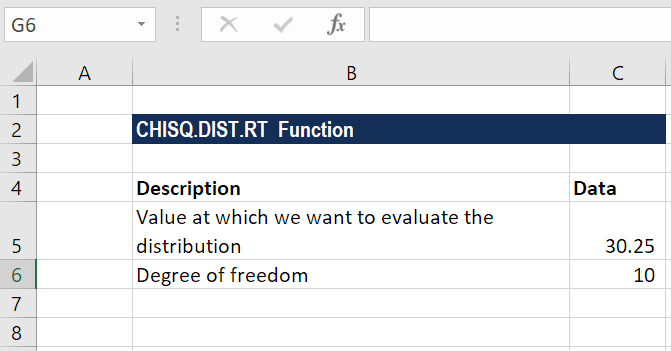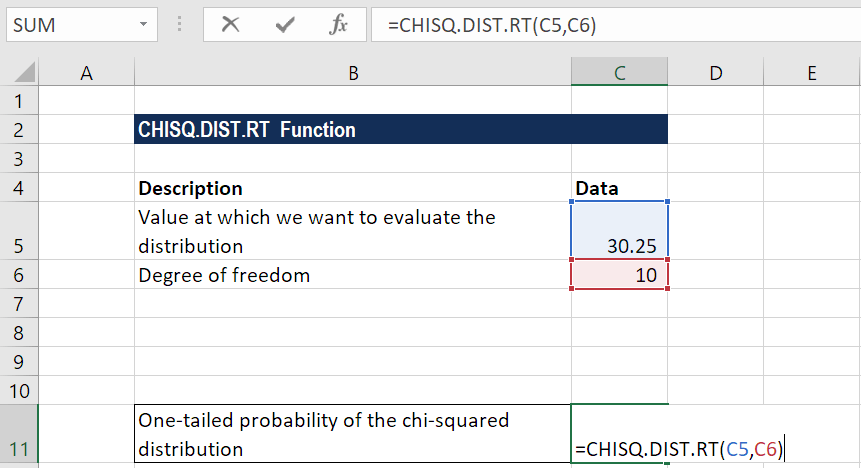# CHISQ.DIST.RT Function

Calculates the right-tailed probability of a chi-square distribution

## What is the CHISQ.DIST.RT Function?

The CHISQ.DIST.RT Function is categorized under Excel Statistical functions. It will calculate the right-tailed probability of a chi-square distribution. We use this function to compare observed and expected values. So, the χ2 distribution is associated with an χ2 test.

In financial analysis, the function can be used to find out and compare observed and expected values. For example, we can find a link between our revenues and the geographical location of our customers.

If we get a small value from the chi-square table, it means that there is not much of a relationship between two given variables. However, if we get a larger value, it indicates a greater relationship between the two variables.

### Formula

=CHISQ.DIST.RT(x,deg_freedom)

The CHISQ.DIST.RT function uses the following arguments:

1. X (required argument) – This is the value at which the chi-square distribution is to be evaluated. It should be greater than or equal to zero.
2. Deg_freedom (required argument) – This is the number of degrees of freedom. It must be an integer between 1 and 1010.

### How to use the CHISQ.DIST.RT Function in Excel?

To understand the uses of CHISQ.DIST.RT function, let us consider an example:

#### Example

Suppose we are given the following data:The formula for calculating the one-tailed probability of the chi-squared distribution is:We get the result below:

### A few notes about the CHISQ.DIST.RT Function

1. If the deg_freedom argument is not an integer, it is truncated by MS Excel.
2. The function belongs to the CHISQ group of functions. It was introduced in MS Excel 2010 and hence is unavailable for earlier versions. The CHISQ.DIST.RT function is an updated version of the CHIDIST function.
3. #VALUE! error – Occurs when any of the arguments provided is non-numeric. Remember, CHISQ.DIST.RT will accept only numeric datasets for both arguments and return numeric data.
4. #NUM! error – Occurs when:
1. The given value of x is negative.
2. The deg_freedom argument is less than 1 or greater than 1010.

CFI is the official global provider of the FMVA Financial Analyst Certification, designed to transform anyone into a world-class financial analyst.

Thank you for reading this guide to important Excel functions and formulas! By taking the time to learn and master these functions, you’ll significantly speed up your financial modeling To learn more, check out these additional CFI resources:

• Excel used for Finance
• Professional Excel Course
• Difficult Excel Formulas for Professionals
• Excel Shortcuts on a Keyboard

### Free Excel Tutorial

To master the art of Excel, check out CFI’s FREE Excel Crash Course, which teaches you how to become an Excel power user.  Learn the most important formulas, functions, and shortcuts to become confident in your financial analysis.

Launch CFI’s Free Excel Course now to take your career to the next level and move up the ladder!